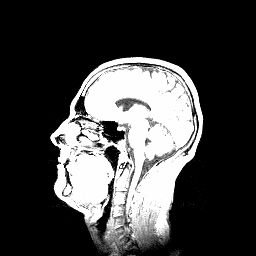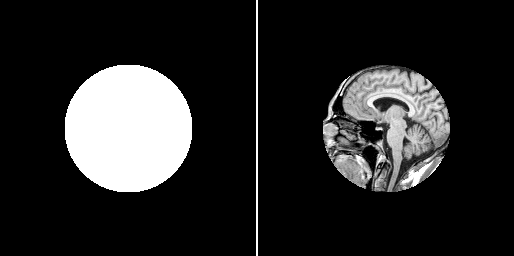# Simple image math and saturation arithmetic¶

This example demonstrates how to do simple mathematical operations on images. All the operations are saturating, i.e. out-of-bounds results are clamped to the allowable value range of the pixel data type instead of wrapping around

Some operations support input images of different size. In this case, out-of-bounds pixels are taken from the nearest valid location in the edge of the image. As a result, one can e.g. multiply each slice of a 3D image by a 2D image. This process is often used to mask bad regions away (e.g. regions outside of the completely reconstructed cylinder in a tomographic image).

def math():
"""
Demonstrates use of simple image math.
"""

# Subtract images
pi.subtract(img, img)

# The math operations are saturating, i.e. if the result of an operation is out of
# bounds that can be represented with pixel data type, the value is clipped
# to the bounds.
# For example,
# 200 + 200 = 255 for uint8 image,
# 200 + 200 = 400 for uint16 image,
# 2*30000 = 65535 for uint16 image,
# etc.

# Do you have a 2D mask that you would like to apply to
# all slices of a 3D stack?
# No problem, just specify True for 'allow dimension broadcast' parameter:

# Create mask whose size is the same than the size of the original but it contains
# only one slice. Then draw a circle into it, with color 1.
pi.sphere(mask, [img.get_width() / 2, img.get_height() / 2, 0], img.get_width() / 4, 1)One slice of the input image. Grayscale range is $$[0, 463]$$.Saturated output image, input image + 65400. Notice that many regions are saturated to white, corresponding to the maximum value of the pixel data type. Grayscale range is $$[65400, 65535]$$.